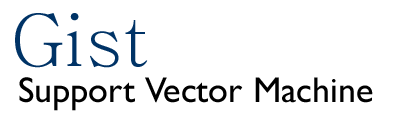## Frequently asked questions

Some of these questions apply primarily to the command line tools.

1. I am using Gist with some data where the negatives are significantly fewer than the positives. Also, I care more about minimizing the false positive rate than the false negative rate. I used the hold-one-out setting and I am getting around 30% FPR, while 12% FNR, using a dot-product in the power of 3 as kernel. I tried also radial as well with no better results. I was thinking that if I was able to move the discriminating plane closer to the positives I would get a better FPR (and possibly a worse FNR, but I care less about that). Since I don't really understand very well how SVM work I am not sure how to do this.

You've got the right idea. What you want is an asymmetric soft margin, which charges more for false positives than for false negatives. Unfortunately, Gist does not allow an asymmetric soft margin in conjunction with the default, 2-norm soft margin. However, you can try using an asymmetric 1-norm soft margin by using `-diagfactor 0` and the `-posconstraint` and `-negconstraint` options to `gist-train-svm.` Try it first using `-posconstraint 1` and `-negconstraint 1`, to see if changing from 1-norm to 2-norm makes a big difference. Then, if you want to penalize false positives more heavily, you can set a smaller constraint on the positive support vectors. So, for example, you might try `-posconstraint 0.5 -negconstraint 1.0`.

An alternative (and equally valid) approach would be to fit a curve using the `gist-sigmoid` script, and then use the resulting probabilities to choose a classification threshold that is not at 50%. In other words, you may want to predict that something is positive only if the SVM gives it a probability of 75% or better. Note that using `gist-sigmoid` properly requires that you train the SVM on a subset of your data (usually about 90%) and then fit the sigmoid using a different set of data (the remaining 10%).

2. How does Gist deals with missing values?

Your data files cannot have any missing values. If we allowed missing values, then we would have to impute them, because the SVM needs complete feature vectors. We prefer to leave the imputation up to the user. A simple way for you to do this is to put in the mean of a feature for all missing values for that feature. An alternative is to skip features that have missing values. In our own work we use a combination of skipping features that have too many missing values (say, >10 or 20%) and imputing values for the rest.

3. I am running Gist and I get the message "Negative delta". What does this mean?

The message "Negative delta" is issued when the size of the margin from one iteration to the next decreases, rather than increases. The results of the optimization will still be valid, though they will be slightly non-optimal. Usually, the effect is very small. To see how bad the problem is, try running `gist-train-svm` with the `-verbose` option set to 3. This will show you the delta at each iteration. In a normal optimization, you will see it get smaller and smaller, until it eventually drops below the convergence threshold. Sometimes, this final value is slightly negative, in which case the "Negative delta" warning message is issued.

4. I got the error message "Possible underflow in radial_kernel", but my run completes normally. What does that mean?

This message can be ignored and will be removed from future versions of Gist. During calculation of radial kernel values, if two examples are very far away from each other, then when you take the exponential of their negative distance, the resulting number can become zero (due to round-off). This error message warns that this has happened, but it is not going to affect the results.

5. If I use kernel-pca with the default dot-product kernel, is that the same as a standard principal component analysis?

Yes.

6. I am getting different results on repeated runs of gist-train-svm.

Most likely the SVM solution isn't being allowed to converge tightly enough. You should try making the `threshold` smaller (try 10e-10). This situation is most common when you are training on small numbers of examples. Note that this will tend to increase the time it takes the SVM to converge.

7. I want to use a kernel function that isn't provided by Gist.

Use the `-matrix` option. This indicates that the file supplied by `-train` is a kernel matrix. This means that you can implement your own kernel function, apply it to your data in a separate step, and have Gist use the resulting kernel matrix as an input. See the documentation of gist-train-svm for details.